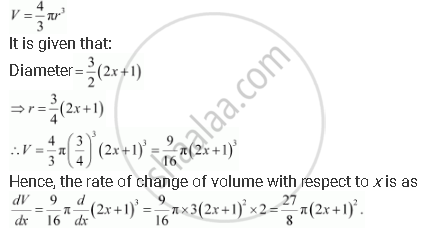Share

# A Balloon, Which Always Remains Spherical, Has a Variable Diameter 3/2 (2x + 1) Find the Rate of Change of Its Volume with Respect To X. - CBSE (Commerce) Class 12 - Mathematics

ConceptRate of Change of Bodies Or Quantities

#### Question

A balloon, which always remains spherical, has a variable diameter  3/2 (2x +   1) Find the rate of change of its volume with respect to x.

#### Solution

The volume of a sphere (V) with radius (r) is given by,Is there an error in this question or solution?

#### APPEARS IN

NCERT Solution for Mathematics Textbook for Class 12 (2018 to Current)
Chapter 6: Application of Derivatives
Q: 13 | Page no. 198

#### Video TutorialsVIEW ALL 

Solution A Balloon, Which Always Remains Spherical, Has a Variable Diameter 3/2 (2x + 1) Find the Rate of Change of Its Volume with Respect To X. Concept: Rate of Change of Bodies Or Quantities.
S